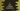# 2 different C programs to print a triangle of prime numbers## C program to print a triangle of prime numbers:

This post will show you how to print a triangle or prime numbers in C programming. It will be a triangle pattern and the program will take the height of the triangle as an input from the user. I will show you different ways to print the pattern.

### Algorithm to print a prime number triangle:

The program will use the below algorithm to print the triangle:

• Take the height as input from the user.
• Run two for loops. The outer loop will run for height number of time. The inner loop will run for the current row number of times. For the first row, it will run for one time, two times for the second row, three times for the third row etc.
• Inside the inner loop, call a method to get the next prime number.

• This method will find the next prime number i.e. the prime number after the last printed prime number.
• It will use one loop to find the next prime number.
• It will keep track of the current prime number that it returned.
• There will be one more method to check if a number is prime or not. This method will iterate from 2 to number/2. It will not be a prime number if any number in the loop can divide it. Else, it will be a prime number.
• At the end of each iteration of the outer loop, it will print one new-line character to move to the next line.

### Example 1: C program to print prime number triangle by using for loops:

Below is the complete C program that prints a triangle of prime numbers:

``````#include <stdio.h>
#include <math.h>
#define False 0;
#define True 1;

int isPrime(int number)
{
for (int i = 2; i <= number / 2; i++)
{
if (number % i == 0)
{
return False;
}
}
return True;
}

int getNextPrime(int currentPrime)
{
currentPrime++;

while (!isPrime(currentPrime))
{
currentPrime++;
}
return currentPrime;
}

int main()
{
int height;
int currentPrime = 1;

printf("Enter the height of the triangle: ");
scanf("%d", &height);

for (int i = 1; i <= height; i++)
{
for (int j = 1; j <= i; j++)
{
currentPrime = getNextPrime(currentPrime);
printf("%-4d ", currentPrime);
}
printf("\n");
}
}``````

Here,

• It asks the user to enter the height of the triangle. It reads the value and assign it to the height variable.
• The currentPrime variable is used to store the current prime value to print. It is initialized as 1.
• The two for loops are used to print the triangle. Inside the inner loop, it calls getNextPrime method to get the next prime number. It passes the value of currentPrime to this method and the return value is assigned to currentPrime.
• The value of currentPrime is printed on each iteration.

• The getNextPrime method is used to get the next prime number. It accepts one number as the parameter and returns the next prime number. The while loop is used to find the next prime number. It calls the isPrime method to check if a value is prime or not. Once it finds a prime value, it returns that.
• The isPrime method is used to check if a number is prime or not. It uses a for loop to iterate from 2 to number/2. The number is not a prime number if any value in the loop can divide it. Else, it is a prime number. If it is prime, it returns 1. Else, it returns 0.

If you run this program, it will print outputs as below:

``````Enter the height of the triangle: 6
2
3    5
7    11   13
17   19   23   29
31   37   41   43   47
53   59   61   67   71   73

Enter the height of the triangle: 10
2
3    5
7    11   13
17   19   23   29
31   37   41   43   47
53   59   61   67   71   73
79   83   89   97   101  103  107
109  113  127  131  137  139  149  151
157  163  167  173  179  181  191  193  197
199  211  223  227  229  233  239  241  251  257  ``````

### Example 2: C program to print prime number triangle by using while loops:

We can also use while loops to print a prime number triangle. Let’s change the above example to use while loops:

``````#include <stdio.h>
#include <math.h>
#define False 0;
#define True 1;

int isPrime(int number)
{
for (int i = 2; i <= number / 2; i++)
{
if (number % i == 0)
{
return False;
}
}
return True;
}

int getNextPrime(int currentPrime)
{
currentPrime++;

while (!isPrime(currentPrime))
{
currentPrime++;
}
return currentPrime;
}

int main()
{
int height;
int currentPrime = 1, i = 1, j;

printf("Enter the height of the triangle: ");
scanf("%d", &height);

while (i <= height)
{
j = 1;
while (j <= i)
{
currentPrime = getNextPrime(currentPrime);
printf("%-4d ", currentPrime);
j++;
}
printf("\n");
i++;
}
}``````

It will print similar outputs. The variables i and j are used in the while loops.

``````Enter the height of the triangle: 5
2
3    5
7    11   13
17   19   23   29
31   37   41   43   47  ``````